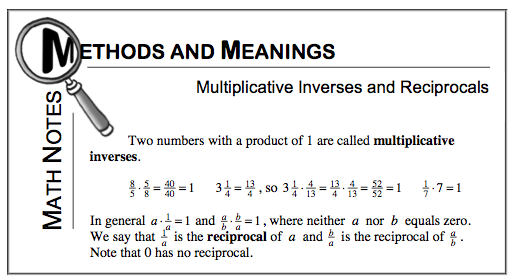Home > CC1 > Chapter 7 > Lesson 7.2.3 > Problem7-65

7-65.

Find the multiplicate inverse of each of the following numbers. Refer to the Math Notes box in this lesson for help. Homework Help ✎

For parts (a) through (d), use the Math Notes box at the bottom of the page as a reference.

1. $\frac { 7 } { 8 }$

$\frac{8}{7}$

1. $1\frac { 2 } { 3 }$

For help, see the Math Notes box below.

1. $1.5$

For help, see the Math Notes box below.

1. $0.25$

4